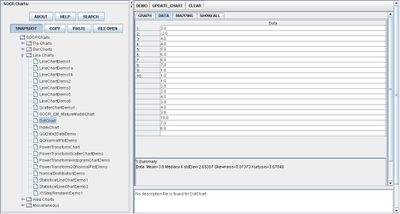Difference between revisions of "SOCR EduMaterials Activities DotChart"

Summary

This activity describes the need, general methods and SOCR utilities for the dot cart.

Goals

The aims of this activity is to:

• increase user's involvement with SOCR utilities such as the dot chart
• create a better understanding of the dot chart for users
• practice skills developed from obtaining knowledge of SOCR dot chart

Background

For continuous, quantitative, univariate data, Dot Charts or Dot Plots are often useful to plot statistical values. By these visualizations, clusters, gaps, and outliers can be easily attainable. But Dot Charts are most helpful for small sets of data whereas stem plots, box plots, or histograms are more efficient for large sets of data.

For references, visit Wikipedia and Definition.

Description

Go to the SOCR Charts and select Line Charts from the items located on the left, then select the demonstration for Dot Charts:The image above is a demonstration of a simple Dot Chart. Note that there is no true vertical axis as it is not labeled but the horizontal axis represents the data. If more than one value of data appears, the data points do not overlap. Instead, they are plotted above the data point of equal value.

Below the graph is a statistical representation of the data values as shown.

Examples & Exercise

• Exercise 1: Because the dot chart can illustrate statistical values by illustration, name some of these information that users can calculate.
• Exercise 2: Does it matter if variables or events being used are independent of dependent?
• Exercise 3: Look into SOCR histogram charts and convert it into a dot chart.

Data Type and Format

By clicking the Data tab (in between the Graph and Mapping), the user may input new or change the values of the existent data set. There is one type of data allowed for Dot Charts and those obtain numerical values. As shown in the image below, the values are simply listed as integers in no particular order:Applications

One of the most persuasive elements when proposing data and literature to others is a well-designed chart presentation.

For example, students in labs may want to present their table of values in a graph. By using the Dot Charts, all the data points are easily represented as well as the frequency of each data value.

Suppose real estate agents want to easily represent the number of bedrooms in the different designs of houses on a property.